# Infinite Expressions for PiThis page lists a number of infinite expressions of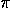. Proofs are not provided here, but the viewer is encouraged to study the sources listed in the reference page.

• John Wallis (1655) took what can now be expressed as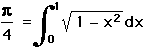and without using the binomial theorem or integration (not invented yet) painstakingly came up with a formula forto be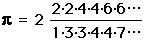.

• William Brouncker (ca. 1660's) rewrote Wallis' formula as a continued fraction, which Wallis and later Euler (1775) proved to be equivalent. It is unknown how Brouncker himself came up with the continued fraction,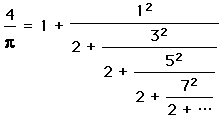.

• James Gregory (1671) & Gottfried Leibniz (1674) used the series expansion of the arctangent function,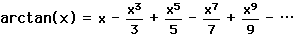,

and the fact that arctan(1) =/4 to obtain the series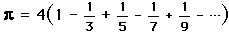.

Unfortunately, this series converges to slowly to be useful, as it takes over 300 terms to obtain a 2 decimal place precision. To obtain 100 decimal places of, one would need to use at least 10^50 terms of this expansion!

• History books credit Sir Isaac Newton (ca. 1730's) with using the series expansion of the arcsine function,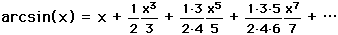,

and the fact that arctan(1/2) =/6 to obtain the series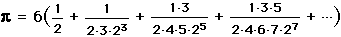.

This arcsine series converges much faster than using the arctangent. (Actually, Newton used a slightly different expansion in his original text.) This expansion only needed 22 terms to obtain 16 decimal places for.

• Leonard Euler (1748) proved the following equivalent relations for the square of,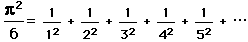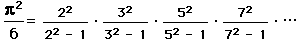• Ko Hayashi (1989) found another infinite expression forin terms of the Fibonacci numbers,.http://www.geom.umn.edu/~huberty/math5337/groupe/expresspi.html